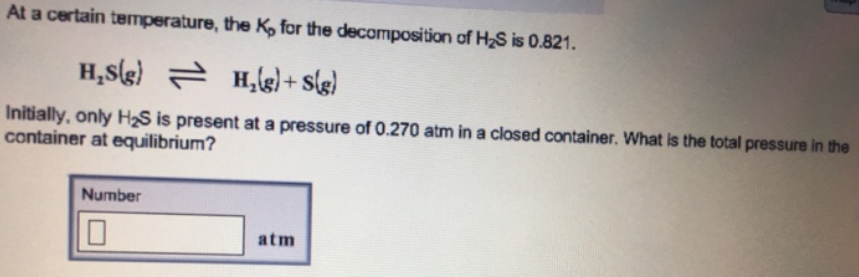# Problem: At a certain temperature, the Kp for the decomposition of H2S is 0.821. H2S (g) ⇌ H2 (g) + S (g) Initially, only H2S is present at a pressure of 0.270 atm in a closed container. What is the total pressure in the container at equilibrium?

🤓 Based on our data, we think this question is relevant for Professor Golde's class at PITT.

###### FREE Expert Solution###### Problem Details

At a certain temperature, the Kp for the decomposition of H2S is 0.821.

H2S (g) ⇌ H2 (g) + S (g)

Initially, only H2S is present at a pressure of 0.270 atm in a closed container.

What is the total pressure in the container at equilibrium?